You will find Chapter 3 Understanding Quadrilaterals NCERT Solutions for Class 8 Maths on this page which will be helpful in finding accurate and detailed NCERT Solutions for Class 8. These are prepared by Studyrankers experts who have vast experience in teaching students. These NCERT Solutions are updated according to the latest pattern of CBSE. You can also download PDF of Chapter 3 Understanding Quadrilaterals NCERT Solutions which help you in achieving more marks in the examinations.

These NCERT Solutions are updated as per the latest syllabus of Class 8 NCERT textbooks. Chapter 3 Class 8 NCERT Solutions can be used for completing your homework or understanding the basic concepts to solve problems.Study Materials for Class 8 Maths Chapter 3 Understanding Quadrilaterals

Page No: 41

Exercise 3.1

1. Given here are some figures.Classify each of them on the basis of the following.
(a) Simple curve       (b) Simple closed curve       (c) Polygon
(d) Convex polygon         (e) Concave polygon

(a) Simple curve: 1, 2, 5, 6 and 7
(b) Simple closed curve: 1, 2, 5, 6 and 7
(c) Polygon: 1 and 2
(d) Convex polygon: 2
(e) Concave polygon: 1

2. How many diagonals does each of the following have?
(a) A convex quadrilateral         (b) A regular hexagon        (c) A triangle

(a) A convex quadrilateral: It has 2 diagonals.(b) A regular hexagon(c) A triangle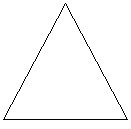3. What is the sum of the measures of the angles of a convex quadrilateral? Will this property hold if the quadrilateral is not convex? (Make a non-convex quadrilateral and try!)Let ABCD be a convex quadrilateral. We observe that the quadrilateral ABCD formed by two triangles i.e. ΔADC and ΔABC.
Since, we know that sum of interior angles of triangle is 180°. Thus, the sum of the measures of the angles is 180° + 180° = 360°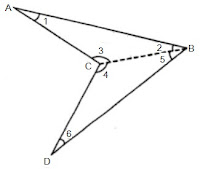Let us take another quadrilateral ABCD which is not convex and join BC which divides it into two triangles ΔABC and ΔBCD.
In ΔABC,
∠1 + ∠2 + ∠3 = 180°   (angle sum property of triangle)
In ΔBCD,
∠4 + ∠5 + ∠6 = 180°   (angle sum property of triangle)
Therefore,  ∠1 + ∠2 + ∠3 + ∠4 + ∠5 + ∠6 = 180° + 180°
⇒ ∠1 + ∠2 + ∠3 + ∠4 + ∠5 + ∠6 = 360°
⇒ ∠A + ∠B + ∠C + ∠D = 360°
Thus, this property hold if the quadrilateral is not convex.

4. Examine the table. (Each figure is divided into triangles and the sum of the angles deduced from that.)What can you say about the angle sum of a convex polygon with number of sides?
(a) 7            (b) 8            (c) 10             (d) n

The angle sum of a polygon having side n = (n-2)×180°
(a) 7
Here, n = 7
Thus, angle sum = (7-2)×180° = 5×180° = 900°

(b) 8
Here, n = 8
Thus, angle sum = (8-2)×180° = 6×180° = 1080°

(c) 10
Here, n = 10
Thus, angle sum = (10-2)×180° = 8×180° = 1440°

(d) n
Here, n = n
Thus, angle sum = (n-2)×180°

Page No: 42

5. What is a regular polygon?
State the name of a regular polygon of
(i) 3 sides          (ii) 4 sides           (iii) 6 sides

A polygon having sides of equal length and angles of equal measures is called regular polygon.
(i) A regular polygon of 3 sides is equilateral triangle.
(ii) A regular polygon of 4 sides is square.
(iii) A regular polygon of 6 sides is regular hexagon.

6. Find the angle measure x in the following figures.(a) The figure is having 4 sides. Hence, it is a quadrilateral.
Sum of angles of the quadrilateral = 360°
⇒ 50° + 130° + 120° + x = 360°
⇒ 300° + x = 360°
⇒ x = 360° - 300° = 60°

(b) The figure is having 4 sides. Hence, it is a quadrilateral. Also, one side is perpendicular forming right angle.
Sum of angles of the quadrilateral = 360°
⇒ 90° + 70° + 60° + x = 360°
⇒ 220° + x = 360°
⇒ x = 360° - 220° = 140°

(c) The figure is having 5 sides. Hence, it is a pentagon.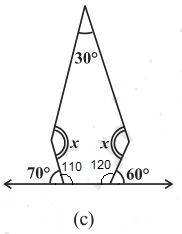Sum of angles of the pentagon = 540°
Two angles at the bottom are linear pair.
Therefore, 180° - 70° = 110°
180° - 60° = 120°
⇒ 30° + 110° + 120° + x + x = 540°
⇒ 260° + 2x = 540°
⇒ 2x = 540° - 260° = 280°
⇒ x = 280°/2 = 140°

(d) The figure is having 5 equal sides. Hence, it is a regular pentagon. Thus, its all angles are equal.
5x = 540°
⇒ x = 540°/5
⇒ x = 108°

7.(a) Sum of all angles of triangle = 180°
One side of triangle = 180°- (90° + 30°) = 60°
x + 90° = 180° ⇒ x = 180° - 90° = 90°
y + 60° = 180° ⇒ y = 180° - 60° = 120°
z + 30° = 180° ⇒ z = 180° - 30° = 150°
x + y + z = 90° + 120° + 150° = 360°

(b) Sum of all angles of quadrilateral = 360°
One side of quadrilateral = 360°- (60° + 80° + 120°) = 360° - 260° = 100°
x + 120° = 180° ⇒ x = 180° - 120° = 60°
y + 80° = 180° ⇒ y = 180° - 80° = 100°
z + 60° = 180° ⇒ z = 180° - 60° = 120°
w + 100° = 180° ⇒ w = 180° - 100° = 80°
x + y + z + w = 60° + 100° + 120° + 80° = 360°

Page No: 44

Exercise 3.2

1. Find x in the following figures.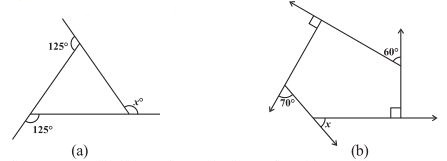(a)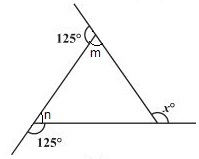125° + m = 180° ⇒ m = 180° - 125° = 55°   (Linear pair)
125° + n = 180° ⇒ n = 180° - 125° = 55°   (Linear pair)
x = m + n (exterior angle of a triangle is equal to the sum of two opposite interior two angles)
⇒ x = 55° + 55° = 110°

(b)Two interior angles are right angles = 90°
70° + m = 180° ⇒ m = 180° - 70° = 110°    (Linear pair)
60° + m = 180° ⇒ m = 180° - 60° = 120°    (Linear pair)
The figure is having five sides and is a pentagon.
Thus, sum of the angles of pentagon = 540°
90° + 90° + 110° + 120° + y = 540°
⇒ 410° + y = 540° ⇒ y = 540° - 410° = 130°
x + y = 180°    (Linear pair)
⇒ x + 130° = 180°
⇒ x = 180° - 130° = 50°

2. Find the measure of each exterior angle of a regular polygon of
(i) 9 sides           (ii) 15 sides

Sum of angles a regular polygon having side n = (n-2)×180°
(i) Sum of angles a regular polygon having side 9 = (9-2)×180°
= 7×180° = 1260°
Each interior angle = 1260°/9 = 140°
Each exterior angle = 180° - 140° = 40°
Or,
Each exterior angle = Sum of exterior angles/Number of sides = 360°/9 = 40°

(i) Sum of angles a regular polygon having side 15 = (15-2)×180°
= 13×180° = 2340°
Each interior angle = 2340°/15 = 156°
Each exterior angle = 180° - 156° = 24°
Or,
Each exterior angle = Sum of exterior angles/Number of sides = 360°/15 = 24°

3. How many sides does a regular polygon have if the measure of an exterior angle is 24°?

Each exterior angle = Sum of exterior angles/Number of sides
24° = 360°/Number of sides
⇒ Number of sides = 360°/24° = 15
Thus, the regular polygon have 15 sides.

4. How many sides does a regular polygon have if each of its interior angles is 165°?

Interior angle = 165°
Exterior angle = 180° - 165° = 15°
Number of sides = Sum of exterior angles/exterior angle
⇒ Number of sides = 360°/15° = 24
Thus, the regular polygon have 24 sides.

5. (a) Is it possible to have a regular polygon with measure of each exterior angle as 22°?
(b) Can it be an interior angle of a regular polygon? Why?

(a) Exterior angle = 22°
Number of sides = Sum of exterior angles/exterior angle
⇒ Number of sides = 360°/22° = 16.36
No, we can't have a regular polygon with each exterior angle as 22° as it is not divisor of 360.

(b) Interior angle = 22°
Exterior angle = 180° - 22°= 158°
No, we can't have a regular polygon with each exterior angle as 158° as it is not divisor of 360.

6. (a) What is the minimum interior angle possible for a regular polygon? Why?
(b) What is the maximum exterior angle possible for a regular polygon?

(a) Equilateral triangle is regular polygon with 3 sides has the least possible minimum interior angle because the regular with minimum sides can be constructed with 3 sides at least..
Since, sum of interior angles of a triangle = 180°
Each interior angle = 180°/3 = 60°

(b) Equilateral triangle is regular polygon with 3 sides has the maximum exterior angle because the regular polygon with least number of sides have the maximum exterior angle possible.
Maximum exterior possible = 180 - 60° = 120°

Page No: 50

Exercise 3.3

1. Given a parallelogram ABCD. Complete each statement along with the definition or property used.DCB = ......
(iii) OC = ......         (iv) m ∠DAB + m ∠CDA = ......

(i) AD = BC   (Opposite sides of a parallelogram are equal)
(ii) ∠DCB = ∠DAB    (Opposite angles of a parallelogram are equal)
(iii) OC = OA    (Diagonals of a parallelogram are equal)
(iv)  m ∠DAB + m ∠CDA = 180°

Page No: 51

2. Consider the following parallelograms. Find the values of the unknowns x, y, z.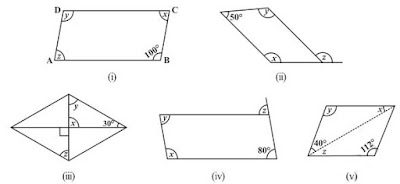(i)y = 100°   (opposite angles of a parallelogram)
x + 100° = 180°   (Adjacent angles of a parallelogram)
⇒ x = 180° - 100° = 80°
x = z = 80°   (opposite angles of a parallelogram)
Thus, x = 80°, y = 100° and y = 100°

(ii)50° + x = 180° ⇒ x = 180° - 50° = 130° (Adjacent angles of a parallelogram)

x = y = 130°   (opposite angles of a parallelogram)
x = z = 130°   (corresponding angle)

(iii)x = 90°   (vertical opposite angles)
x + y 30° = 180°   (angle sum property of a triangle)
⇒ 90° + y 30° = 180°
⇒ y = 180° - 120° = 60°
also, y = z = 60°   (alternate angles)

(iv)z = 80°   (corresponding angle)
z = y = 80°   (alternate angles)
x + y = 180°   (adjacent angles)
⇒ x + 80° = 180° ⇒ x = 180° - 80° = 100°

3. Can a quadrilateral ABCD be a parallelogram if
(i) ∠D + ∠B = 180°?         (ii) AB = DC = 8 cm, AD = 4 cm and BC = 4.4 cm?
(iii) ∠A = 70° and ∠C = 65°?

(i)Yes, a quadrilateral ABCD be a parallelogram if ∠D + ∠B = 180° but it should also fulfilled some conditions which are:
• The sum of the adjacent angles should be 180°.
• Opposite angles must be equal.

(ii) No, opposite sides should be of same length. Here, AD ≠ BC

(iii) No, opposite angles should be of same measures. ∠A ≠ ∠C

4. Draw a rough figure of a quadrilateral that is not a parallelogram but has exactly two opposite angles of equal measure.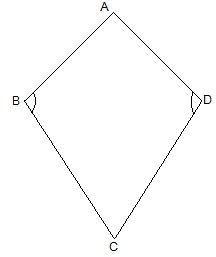ABCD is a figure of quadrilateral that is not a parallelogram but has exactly two opposite angles that is ∠B = ∠D of equal measure. It is not a parallelogram because ∠A ≠ ∠C.

5. The measures of two adjacent angles of a parallelogram are in the ratio 3 : 2. Find the measure of each of the angles of the parallelogram.

Let the measures of two adjacent angles ∠A and ∠B be 3x and 2x respectively in parallelogram ABCD.
∠A ∠B = 180°
⇒ 3x + 2x = 180°
⇒ 5x = 180°
⇒ x = 36°
We know that opposite sides of a parallelogram are equal.
∠A = ∠C = 3x = 3 × 36° = 108°
∠B = ∠D = 2x = 2 × 36° = 72°

6. Two adjacent angles of a parallelogram have equal measure. Find the measure of each of the angles of the parallelogram.

Let ABCD be a parallelogram.
Sum of adjacent angles of a parallelogram =  180°
∠A + ∠B = 180°
⇒ 2∠A = 180°
⇒ ∠A = 90°
also, 90° + ∠B = 180°
⇒ ∠B = 180° - 90° = 90°
∠A = ∠C = 90°
∠B = ∠D = 90°

7. The adjacent figure HOPE is a parallelogram. Find the angle measures x, y and z. State the properties you use to find them.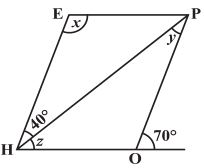y = 40°   (alternate interior angle)
∠P = 70°   (alternate interior angle)
∠P = ∠H = 70°   (opposite angles of a parallelogram)
z = ∠H - 40°= 70° - 40° = 30°
∠H + x = 180°
⇒ 70° + x = 180°
⇒ x = 180° - 70° = 110°

8. The following figures GUNS and RUNS are parallelograms. Find x and y. (Lengths are in cm)(i) SG = NU and SN = GU   (opposite sides of a parallelogram are equal)
3x = 18
⇒ x = 18/3 = 6
3y - 1 = 26 and,
⇒ 3y = 26 + 1
⇒ y = 27/3 = 9
x = 6 and y = 9

(ii) 20 = y + 7 and 16 = x + y   (diagonals of a parallelogram bisect each other)
y + 7 = 20
⇒ y = 20 - 7 = 13 and,
x + y  = 16
⇒ x + 13 = 16
⇒ x = 16 - 13 = 3
x = 3 and y = 13

9. In the above figure both RISK and CLUE are parallelograms. Find the value of x.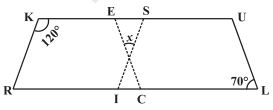∠K + ∠R = 180°   (adjacent angles of a parallelogram are supplementary)
⇒ 120° + ∠R = 180°
⇒ ∠R = 180° - 120° = 60°
also, ∠R = ∠SIL   (corresponding angles)
⇒ ∠SIL = 60°
also, ∠ECR = ∠L = 70°   (corresponding angles)
x + 60° + 70° = 180°   (angle sum of a triangle)
⇒ x + 130° = 180°
⇒ x = 180° - 130° = 50°

Page No: 52

10. Explain how this figure is a trapezium. Which of its two sides are parallel? (Fig 3.32)When a transversal line intersects two lines in such a way that the sum of the adjacent angles on the same side of transversal is 180° then the lines are parallel to each other.
Here, ∠M + ∠L = 100° + 80° = 180°
Thus, MN || LK
As the quadrilateral KLMN has one pair of parallel line therefore it is a trapezium.
MN and LK are parallel lines.

11. Find m∠C in Fig 3.33 if  AB || DC ?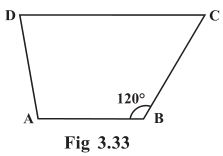m∠C m∠B = 180°   (angles on the same side of transversal)
⇒ m∠C + 60° = 180°
⇒ m∠C = 180°- 60° = 120°

12. Find the measure of ∠P and ∠S if  SP || RQ ? in Fig 3.34. (If you find m∠R, is there more than one method to find m∠P?)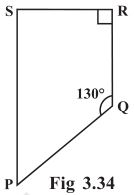∠P ∠Q = 180°   (angles on the same side of transversal)
⇒ ∠P + 130° = 180°
⇒ ∠P = 180° - 130° = 50°
also, ∠R + ∠S = 180°   (angles on the same side of transversal)
⇒ 90° + ∠S = 180°
⇒ ∠S = 180° - 90° = 90°
Thus, ∠P = 50° and ∠S = 90°

Yes, there are more than one method to find m∠P.
PQRS is a quadrilateral. Sum of measures of all angles is 360°.
Since, we know the measurement of ∠Q, ∠R and ∠S.
∠Q = 130°, ∠R = 90° and ∠S = 90°
∠P + 130° + 90° + 90° = 360°
⇒ ∠P + 310° = 360°
⇒ ∠P = 360° - 310° = 50°

Page No: 55

Exercise 3.4

1. State whether True or False.
(a) All rectangles are squares.
(b) All rhombuses are parallelograms.
(c) All squares are rhombuses and also rectangles.
(d) All squares are not parallelograms.
(e) All kites are rhombuses.
(f) All rhombuses are kites.
(g) All parallelograms are trapeziums.
(h) All squares are trapeziums.

(a) False. All square are rectangles but all rectangles are not square.
(b) True
(c) True
(d) False. All squares are parallelograms as opposite sides are parallel and opposite angles are equal.
(e) False. A kite doesn't have all sides of same length.
(f) True
(g) True
(h) True

2. Identify all the quadrilaterals that have.
(a) four sides of equal length       (b) four right angles

(a) Rhombus and square have all four sides of equal length.
(b) Square and rectangle have four right angles.

3. Explain how a square is.
(i) a quadrilateral    (ii) a parallelogram   (iii) a rhombus    (iv) a rectangle

(i) Square is a quadrilateral because it has four sides.
(ii) Square is a parallelogram because its opposite sides are parallel and opposite angles are equal.
(iii) Square is a rhombus because its all four sides of equal length and diagonals bisect at right angles.
(iv) Square is a rectangle because its each interior angle is 90°

4. Name the quadrilaterals whose diagonals.
(i) bisect each other    (ii) are perpendicular bisectors of each other    (iii) are equal

(i) Parallelogram, Rhombus, Square and Rectangle
(ii) Rhombus and Square
(iii) Rectangle and Square

5. Explain why a rectangle is a convex quadrilateral.

Rectangle is a convex quadrilateral because its both diagonals lie inside the rectangle.

6. ABC is a right-angled triangle and O is the mid point of the side opposite to the right angle. Explain why O is equidistant from A, B and C. (The dotted lines are drawn additionally to help you).AD and DC are drawn so that AD || BC and AB || DC
AD = BC and AB = DC
ABCD is a rectangle as opposite sidesare equal and parallel to each other and all the interior angles are of 90°.
In a rectangle, diagonals are of equal length and also bisects each other.
Hence, AO = OC = BO = OD
Thus, O is equidistant from A, B and C.

Go Back To NCERT Solutions for Class 8 Maths

## NCERT Solutions for Class 8 Maths Chapter 3 Understanding Quadrilaterals

Chapter 3 Understanding Quadrilaterals Class 8 Maths is very helpful in fetching good marks in the examinations. Before going for supplementary you must try to solve these NCERT Solutions which will given you proper understanding of the chapter.

A simple closed curve made up of only line segments is called a polygon. We classify polygons according to the number of sides (or vertices) they have such as Triangles (3 sides), Quadrilaterals (4 sides), Pentagon (5 sides) and so on. We will study about convex and concave,  regular and irregular polygons. After that we will discuss about the types of quadrilaterals like trapezium, kite, parallelogram. Also, we will talk about parallelograms briefly by studying about their angles, diagonals and special parallelograms like rhombus, rectangle, square. NCERT Solutions for Class 8 Maths will improve the learning behaviour of students.

Below you will find exercisewise NCERT Solutions of Chapter 3 Class 8 Maths that are detailed and step by step so one can easily solve their doubts.

### NCERT Solutions for Class 8 Maths Chapters:

 Chapter 1 Rational Numbers Chapter 2 Linear Equations in Variable Chapter 4 Practical Geometry Chapter 5 Data Handling Chapter 6 Square and Square Roots Chapter 7 Cube and Cube Roots Chapter 8 Comparing Quantities Chapter 9 Algebraic Expressions and Identities Chapter 10 Visualizing Solid Shapes Chapter 11 Mensuration Chapter 12 Exponents and Powers Chapter 13 Direct and Inverse Proportions Chapter 14 Factorization Chapter 15 Introduction to Graphs Chapter 16 Playing with Numbers

FAQ on Chapter 3 Understanding Quadrilaterals

#### How many exercises in Chapter 3 Understanding Quadrilaterals

There are 4 exercise in the Chapter 3 Understanding Quadrilaterals which are very useful in completing homework and also when facing doubts.

#### What is equilateral triangle?

An equilateral triangle is a regular polygon of 3 sides and square is a regular polygon of 4 sides.

#### In a quadrilateral ABCD, the angles A, B, C and D are in the ratio 1 : 2 : 3 : 4. Find the measure of each angle of the quadrilateral.

∵∠A : ∠B : ∠C : ∠D = 1 : 2 : 3 : 4
∴ Let us suppose that
∠A = 1x°, ∠B = 2x°

∠C = 3x°, –D = 4x°
Since, ∠A +∠B + ∠C +∠D = 360°
∴ x + 2x + 3x + 4x = 360°
⇒ 10x = 360°
⇒ x = 360/10 = 36°
∴ Angles are:
∠A = x° = 36°
∠B = 2x° = 2 x 36° = 72°
∠C = 3x° = 3 x 36° = 108°
∠D = 4x° = 4 x 36° = 144°

Thus, the measure of the angle of the quad. are 36°, 72°, 108° and 144°

#### The interior angle of a regular is 108°. Find the number of sides of the polygon.

Let there are ‘n’ sides of the regular polygon.
∴ Measure of each exterior angle =  (360/n)o
Since, the measure of each of the interior angle = 108°
∴ Measure of each exterior angle = 180° – 108° = 72°
⇒ 360/n = 72  ⇒ n = 360/72 = 5
Thus, the required number of sides of the regular polygon is 5.